# 乐土助力临床参数与肿瘤突变负荷TMB相关性研究

#### 研究发现

1）对于血液样本，与年龄≤56岁或年龄在56岁~68岁的患者相比，年龄≥68岁的患者TMB明显升高。携带KRAS p.G12突变与KRAS其他变异患者的TMB具有显著差异。

3）无论在组织样本还是血液样本中，TMB和MSI值无线性相关。

#### 总结

TMB可能与年龄、肿瘤类型以及KRAS突变相关。作为免疫检查点抑制剂的一个潜在生物标志物，分析年龄、肿瘤类型和KRAS突变可能为TMB的评估提供更有益的帮助。

### 文章具体解读

##### 分析结果/样本特征

Table 1 Characteristics of blood samples and tissue samples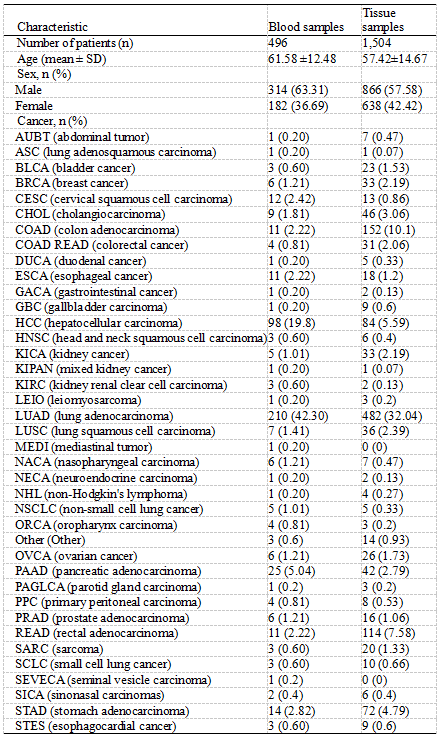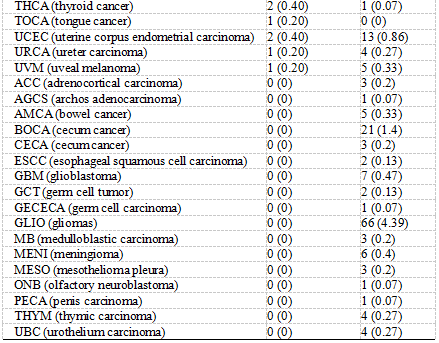##### TMB与年龄、肿瘤类型及基因突变的关系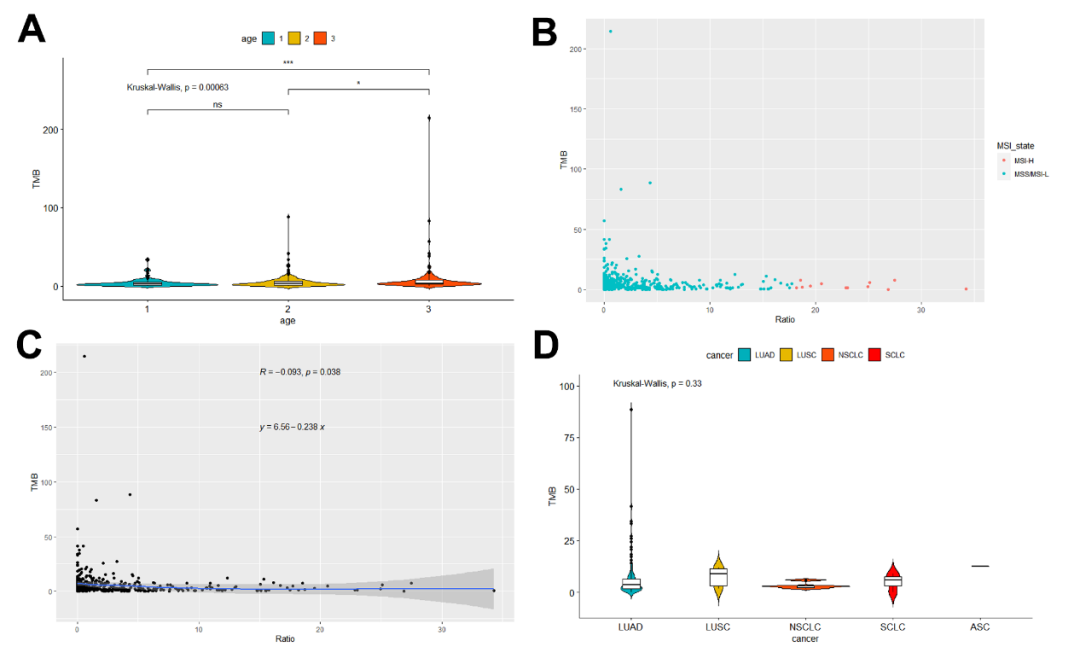A. TMB在年龄≤56岁（n=157），年龄56-68岁（n=177）和年龄≥68岁（n=161）之间的表达差异。

B&C. MSI-H（n=12）和MSS/MSI-L（n=484）的情况及血液样本中MSI比值与TMB的关系。

D. 肺腺鳞癌（n=0）、肺腺癌（n=131）、肺鳞癌（n=7）、非小细胞肺癌（n=3）和小细胞肺癌（n=3）样本中的TMB情况。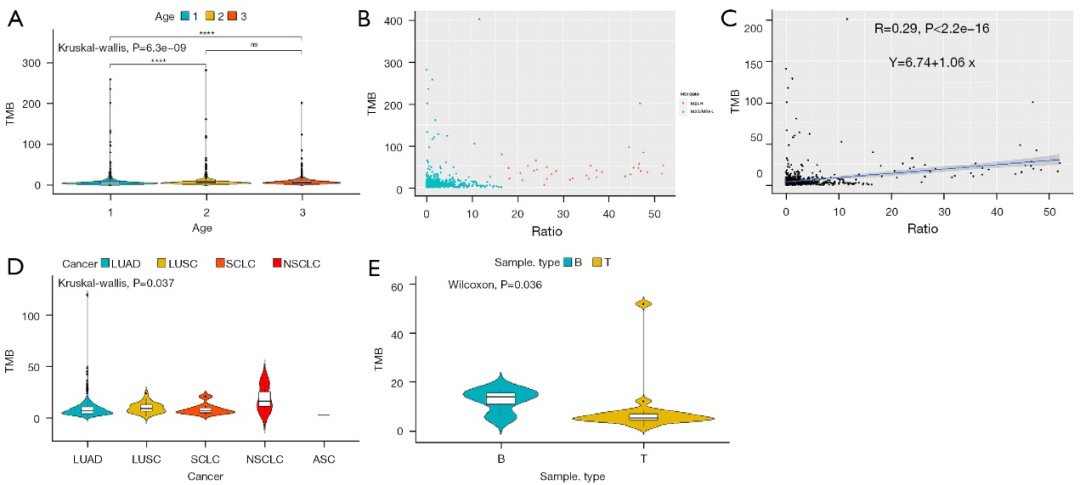A. TMB在年龄≤53岁（n=489），年龄53-65岁（n=497）和年龄≥65岁（n=512）之间的表达差异。

B&C. MSI-H（n=33）和MSS/MSI-L（n=1357）的情况及组织样本中MSI比值与TMB的关系。

D. 肺腺鳞癌（n=1）、肺腺癌（n=222）、肺鳞癌（n=26）、非小细胞肺癌（n=3）和小细胞肺癌（n=8）样本中TMB表达情况。

E. TMB在结直肠癌血液样本（n=4）和组织样本（n=20）中的表达情况。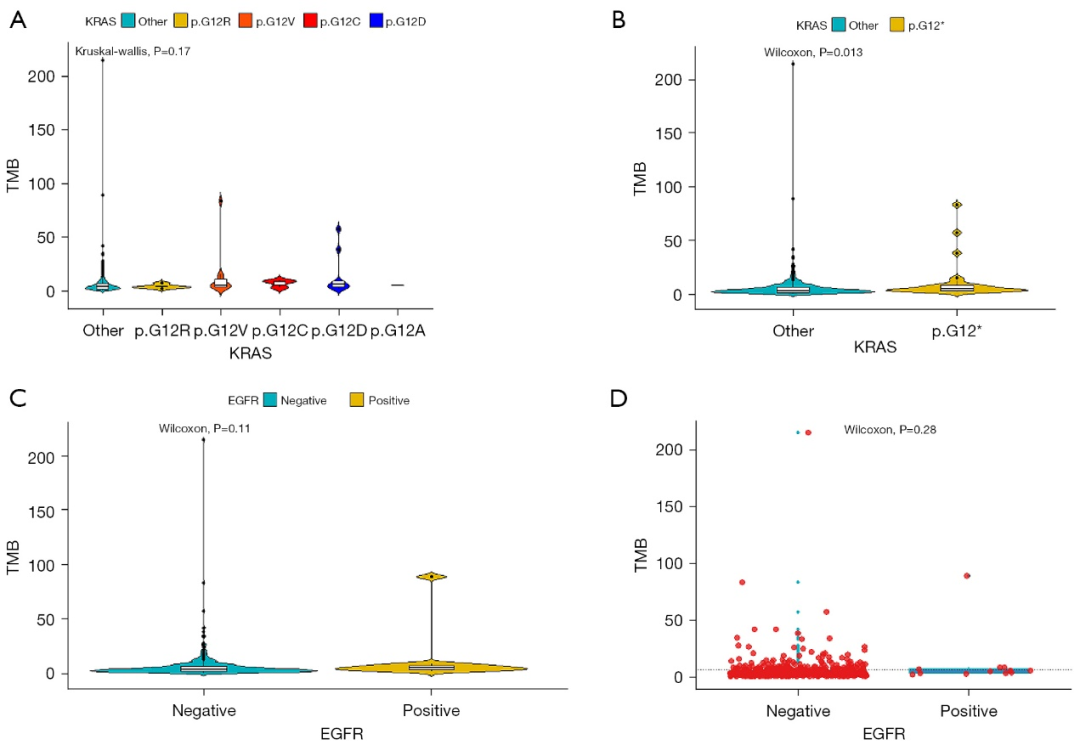A. TMB在KRAS p.G12A (n=1), p.G12C (n=3), p.G12D (n=9), p.G12R (n=5), p.G12V (n=11),和KRAS其他突变 (n=467)中的表达情况。
B. TMB在KRAS其他突变（n=467）和KRAS p.G12（n=29）中的表达情况。
C. TMB在EGFR突变阴性患者 (n=484)和突变阳性患者 (n=12)中的表达情况。
D. TMB在EGFR T790M突变阴性和突变阳性患者之间的表达情况。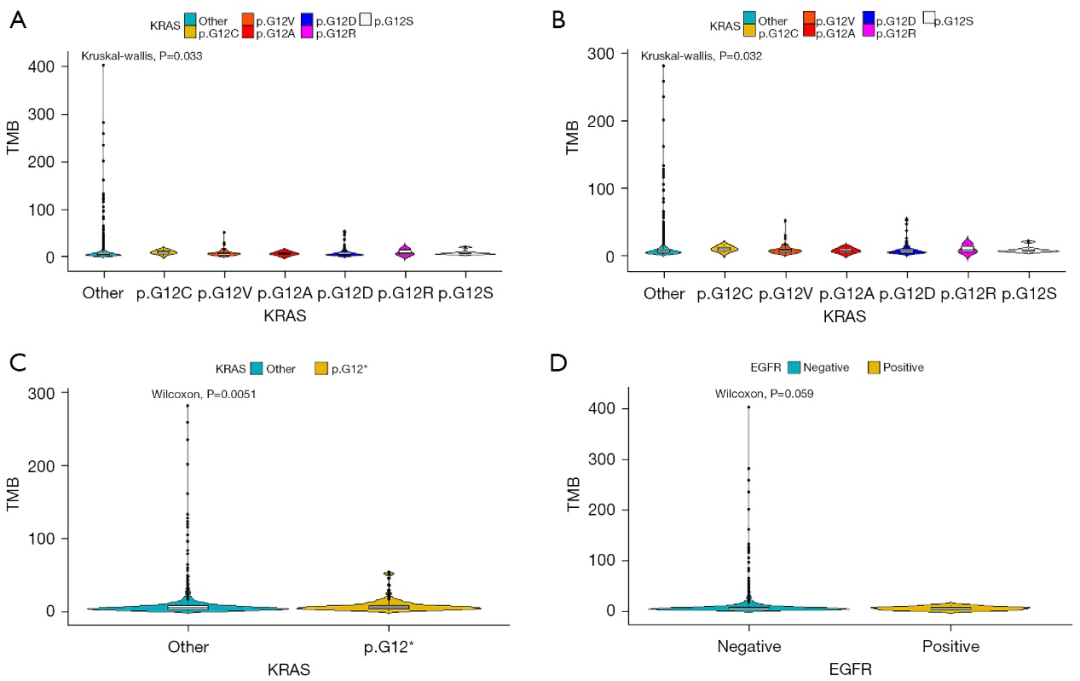A&B. TMB在KRAS p.G12A (n=7), p.G12C (n=13), p.G12D (n=81), p.G12R(n=6), p.G12S(n=8), p.G12V(n=56), 和KRAS其他突变(n=1333)中的表达情况。

C. TMB在KRAS其他突变（n=1333）和KRAS p.G12（n=171）中的表达情况。

D. TMB在EGFR突变阴性患者 (n=1497) 和 EGFR突变阳性患者 (n=7)中的表达情况。